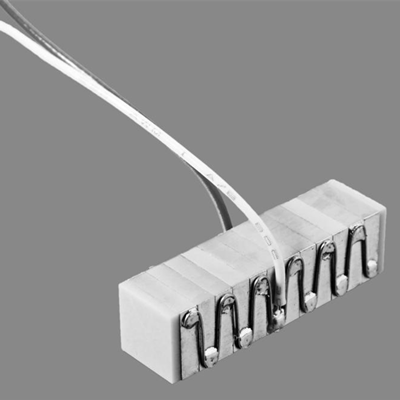Piezo Hannas (WuHan) Tech Co,.Ltd.－Professional Piezoceramic Elements Supplier
News
You are here: / / / Piezoelectric equation of piezoelectric vibrator

# Piezoelectric equation of piezoelectric vibrator

Views:19     Author:Site Editor     Publish Time: 2018-01-21      Origin:SitePiezoelectric equation of piezoelectric vibrator

Piezoelectric ceramics have piezoelectric properties, they exert extra charge when pressure is applied. The piezoelectric effect reflects the coupling between the elastic and dielectric properties of the crystal. Piezoelectric equations reflect the relationship between elastic variables (ie, stress and strain) and electrical variables (ie, electric field and electrical displacement) of pzt piezoelectric transducer piezoelectric ceramics. Thinking of the crystal as a thermodynamic system, starting with the thermodynamic eigenfunction describing the state of the system. According to the theory of thermodynamics, for the reversible process, the micro-change of the internal energy of system can be expressed as (refer to the unit volume)the piezoelectric circuit piezoelectric body, the micro-work done by the outside world to the system includes the elastic force and the micro- Micromotor power made by the micro-work done for the electric field power .Available piezoelectric body can differentiate the expression,Selecting a certain independent variables, differential equations obtained by internal thermodynamic equation of state, which can be the corresponding piezoelectric equation. When the ultrasonic trasducer sensors of piezoelectric vibrator is applied to a resonant filter, a transducer, a delay line and an acousto-optic device, in order to effectively excite the desired vibration mode, the ultrasonic piezo transducer piezoelectric vibrator of a certain crystal material must be selected to have a specific shape and size .And the mode of excitation, that is the piezoelectric vibrators in different vibration modes generally have different shapes and boundary conditions. Therefore, the piezoelectric equations are describing the piezoelectric vibrators are also different. During the experiment, we should determine the vibration mode of the piezoelectric vibrator according to the specific application of the piezoelectric ceramic, that is, selecting the appropriate boundary conditions of the corresponding piezoelectric shape to establish the equation, find the factors that affect the performance, and get the optimal solution. For different boundary conditions for calculation convenience,it often choose different independent variables and dependent variables to describe the piezoelectric oscillator piezoelectric equation.

Feedback
Piezo Hannas (WuHan) Tech Co,.Ltd  is a professional piezoelectric ceramics and ultrasonic transducer manufacturer, dedicated to ultrasonic technology and industrial applications.